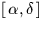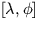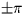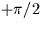Next: SLA_PCD - Apply Radial Distortion
Up: SUBPROGRAM SPECIFICATIONS
Previous: SLA_PA - to Parallactic Angle

## SLA_PAV - Position-Angle Between Two Directions

ACTION:
Returns the bearing (position angle) of one celestial direction with respect to another (single precision).

CALL:
R = sla_PAV (V1, V2)

GIVEN:

 R(3) V1 direction cosines of one point V2 R(3) directions cosines of the other point

RETURNED:

 R sla_PAV position-angle of 2nd point with respect to 1st

NOTES:
1.
The coordinate frames correspond to,etc..
2.
The result is the bearing (position angle), in radians, of point V2 as seen from point V1. It is in the range. The sense is such that if V2 is a small distance due east of V1 the result is about. Zero is returned if the two points are coincident.
3.
The routine sla_BEAR performs an equivalent function except that the points are specified in the form of spherical coordinates.Next: SLA_PCD - Apply Radial Distortion
Up: SUBPROGRAM SPECIFICATIONS
Previous: SLA_PA - to Parallactic Angle

SLALIB --- Positional Astronomy Library